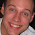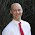# Jeromy Anglim's Blog: Psychology and Statistics

## Monday, September 7, 2009

### Significance Tests on Correlations

OVERVIEW: I often speak to researchers wanting to compare the significance of two correlations. The two scenarios most commonly encountered are: 1) comparing dependent correlations; and 2) comparing independent correlations.

Two dependent correlations
This is the scenario when you have three variables, x, y, and z, and you want to compare the x-y correlation with the x-z correlation. It comes up often when you want to know which of two variables are more related to a third variable. In this sense it is often related to approaches that attempt to assess variable importance using multiple regression.

The main difficulty that most researchers that I talk to have (using SPSS) is that SPSS does not have an built-in tool to test for the statistical significance of a difference between correlations.

In a previous post, I discuss how to run a significance test with R, and in the comments for the post is a link to an SPSS macro that will do the same. For more information about the formulas follow this link.

If you want to obtain confidence intervals of the difference in two dependent correlations, read this.

Two independent correlations.
This is the scenario when two correlations are obtained from different samples and you want to test whether they are significantly different. An example is where a researcher wants to know whether intelligence test scores and performance are correlated the same in different social groups. In most cases, this is very similar to testing for a group by IV interaction effect. Thus, moderator regression is often a more appropriate means of testing the relationship. However, you can also run a specific test of statistical significance on the difference between the two correlations.

Other scenarios include: 3) testing whether a correlation is significantly different from some target value, usually zero, but possibly another value.; 4) a significance test on a correlation matrix; 5) Structural Equation Modelling software can also be used to test more general hypotheses about patterns in correlation matrices. But these things, I'll save for another post.

For scenarios 1, 2, and 3 above, Howell (Statistical Methods for Psychology) sets out the formulas in his chapter on Correlations.

1.What if you wanted to determine if two correlations are significantly different where the two correlations compare the same x/y pairs - one with and one without another variable partialled out? The subjects are the same.

2.I see you've asked the question here:
http://stats.stackexchange.com/questions/9718/appropriate-test-to-determine-if-a-partial-r-between-x-y-is-signficantly-differ

Hopefully that will yield some good responses.

3.You wrote: "An example is where a researcher wants to know whether intelligence test scores and performance are correlated the same in different social groups."
So, using SPSS or R or others, what to do if there are more than two groups? Specifically, the number of groups I have is 6. These are the groups of body height, for instance
< 151cm,
151-160cm,
161-170cm,
171-180cm,
181-190cm,
> 190cm

4.@Rivo In relation to your specific question, you could model your data as a moderator regression.
If you were initially considering looking at how the correlation between X and Y varied over height, you could alternatively run a regression looking at predicting Y from X, height and the X by height interaction. You might also explore quadratic effects of height and even X by quadratic interactions if that made sense.

In particular, you might want to take advantage of the continuous nature of height.

Alternatively, you could plot the correlations for each height group and see if there are any patterns.
You could always apply the above approaches in a pairwise way to test whether a pair of correlations are significantly different.

lists a formula to explicitly tests the null hypothesis that three or more independent correlations are the same.
I'm not sure whether an R implementation of the formula is available.

I asked the question here, so you may get some further suggestions:
http://stats.stackexchange.com/questions/12663/test-in-r-of-whether-three-or-more-correlations-from-independent-samples-are-equa

5.Another case is when you have two different correlations (r12 and r34), but the data is from the same sample. I found out how to calculate the significance test (see http://www.surrey.ac.uk/psychology/current/statistics/), but I'm not sure how to construct confidence intervals for it. I found this calculator (http://faculty.vassar.edu/lowry/VassarStats.html), but I'm not sure if CI's are calculated the same for all types of significance tests between the different types of correlations. Would you happen to know the answer to this question?

6.This comment has been removed by the author.

7.Hi! I am running an experiment on the processing of different word orders (verb-subject-object) and (subject-verb-object) and my findings show that the mean times for females versus males are significantly different. How can I run the significance test for those? And would it be considered as consisting of 3 or 4 variables?

1.I don't think that has much to do with correlations. I suggest you ask a question on http://stats.stackexchange.com
You'll probably get a good answer there.

8.any way to test if a correlation coefficient is or is not significantly different from 1? In other words, the null hypothesis is correlation=1?

9.At the very least your sample correlation needs to be 1.0. A single observation that does not fall on the line of best fit is enough to show that the population correlation is not 1.0.

Alternatively, you might be comparing models that suggest that the correlation is close to 1 with one where the correlation is some other value. In that case, you could do various things. You could compute a Bayesian credibility interval and see whether a sufficient amount of it is above a certain value. You could test whether the correlation is greater than some value e.g., r>.90 or >.95, or >.99.

10.One question that I have not seen addressed when comparing two independent correlations, is whether either or both of the coefficients have to be significantly different from zero. For example, for one sample, the correlation coefficient between two variables might be +.30, and for the other sample, the coefficient might be -.30. Neither are significantly different from zero, but given the sample sizes, they could be different from each other. Is this legitimate?

1.Yes. That is legitimate. Two correlations may be significantly different from one another but one or both may not be significantly different from zero.

I think this may appear strange to some people because people sometimes incorrectly interpret failure to reject the null hypothesis to mean that the null hypothesis is true.

2.11.Hi there,

I'm wondering about how to analyze and interpret differences between correlations of two measures at pre-test versus post-test for a single sample. For example, before a week-long intervention, I find that state-measures of X and Y are significantly correlated, but after intervention they are no longer correlated. How would I test if there was a significant change happening, and how to make sense of that?

12.This situation is discussed here under "nonoverlapping correlations":

Raghunathan, TE, Rosenthal, R. & Rubin, D.B. (1996). Comparing correlated but nonoverlapping correlations.. Psychological Methods, 1, 178.

There also seems to be a blog post implementing a function in R for comparing non-overlapping correlations:
http://seriousstats.wordpress.com/2012/02/05/comparing-correlations/

1.Thank you so very much for your help! My sincere gratitude.

13.Hello,

I am interested in testing three dependent correlations, such that H0: ρ_12=ρ_13=ρ_14. How can I generalize the case of two dependent correlations to three?

Thanks.

14.One approach would be to use SEM software like Amos. (a) standardise all variables; (b) compare a model with and without the three correlations constrained to be equal.

15.Hello, I have use the model to get two series of daily correlation between A and B,(series one is of time 2001-2002, series two is of time 2003-2005) how can I prove the daily correlation of series one is significant different from the daily correlation of series two? should I reduce Number of data in series two to the same amount as in series one?
Thank you very much!

16.Hi,

I have use model to get two series of daily correlation, (these two series are of different time period, series one--2001 to 2002, series two--2003-2005), how could I prove daily correlation of series one is significant from daily correlation of series two?? should I cut down the number of data in series two to the same amount as series one??

Thanks

1.I'm assuming you are asking whether the correlation between one pair of time series is greater than another pair of time series. I'd post the question here
http://stats.stackexchange.com/

as time series is not my area of expertise.

I imagine the length of the time series would influence a few things. (1) more data points means greater precision in estimation if estimating the correlation of the underlying data generating mechanism (2) correlations in time series can be influenced by a range of factors from short term fluctuations to long term fluctuations. Thus, I imagine comparing time series of different durations, correlations might be different due to that factor.

2.Hi there,
In my data, the different correlations are not statistically significant, is it still appropriate to compare the correlation coeffiecients?

17.Hello, I want to present a correlation table with correlations among women and men seperately. I also want to include in this table the significance tests on correlations. Do you have a recommendation how to visually include it in the table? I haven't seen it in articles.